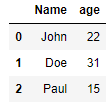1612354800

# How to Rename Pandas DataFrame Column in Python

Pandas is a Python library for data analysis and manipulation. Almost all operations in `pandas` revolve around `DataFrame`s.

`Dataframe` is is an abstract representation of a two-dimensional table which can contain all sorts of data. They also enable us give all the columns names, which is why oftentimes columns are referred to as attributes or fields when using `DataFrames`.

In this article we’ll see how we can rename an already existing `DataFrame`’s columns.

There are two options for manipulating the column names of a `DataFrame`:

1. Renaming the columns of an existing `DataFrame`
2. Assigning custom column names while creating a new `DataFrame`

Let’s take a look at both of the methods.

#### Renaming Columns of an Existing Dataframe

We have a sample `DataFrame` below:

``````import pandas as pd
data = {'Name':['John', 'Doe', 'Paul'],
'age':[22, 31, 15]}
df = pd.DataFrame(data)
``````

The `DataFrame` `df` looks like this:#python #pandas #tool

## Buddha Community1619518440

## top 30 Python Tips and Tricks for Beginners

Welcome to my Blog , In this article, you are going to learn the top 10 python tips and tricks.

### 8) Check The Memory Usage Of An Object.

#python #python hacks tricks #python learning tips #python programming tricks #python tips #python tips and tricks #python tips and tricks advanced #python tips and tricks for beginners #python tips tricks and techniques #python tutorial #tips and tricks in python #tips to learn python #top 30 python tips and tricks for beginners1624431580

## How to add a new column to Pandas DataFrame?

In this tutorial, we are going to discuss different ways to add a new column to pandas data frame.

### What is a pandas data frame?

Pandas data frameis a two-dimensional heterogeneous data structure that stores the data in a tabular form with labeled indexes i.e. rows and columns.

Usually, data frames are used when we have to deal with a large dataset, then we can simply see the summary of that large dataset by loading it into a pandas data frame and see the summary of the data frame.

In the real-world scenario, a pandas data frame is created by loading the datasets from an existing CSV file, Excel file, etc.

But pandas data frame can be also created from the listdictionary, list of lists, list of dictionaries, dictionary of ndarray/lists, etc. Before we start discussing how to add a new column to an existing data frame we require a pandas data frame.

#pandas #dataframe #pandas dataframe #column #add a new column #how to add a new column to pandas dataframe1619510796

## Lambda, Map, Filter functions in python

Welcome to my Blog, In this article, we will learn python lambda function, Map function, and filter function.

Lambda function in python: Lambda is a one line anonymous function and lambda takes any number of arguments but can only have one expression and python lambda syntax is

Syntax: x = lambda arguments : expression

Now i will show you some python lambda function examples:

#python #anonymous function python #filter function in python #lambda #lambda python 3 #map python #python filter #python filter lambda #python lambda #python lambda examples #python map

1586702221

## Python Pandas Objects - Pandas Series and Pandas Dataframe

In this post, we will learn about pandas’ data structures/objects. Pandas provide two type of data structures:-

### Pandas Series

Pandas Series is a one dimensional indexed data, which can hold datatypes like integer, string, boolean, float, python object etc. A Pandas Series can hold only one data type at a time. The axis label of the data is called the index of the series. The labels need not to be unique but must be a hashable type. The index of the series can be integer, string and even time-series data. In general, Pandas Series is nothing but a column of an excel sheet with row index being the index of the series.

### Pandas Dataframe

Pandas dataframe is a primary data structure of pandas. Pandas dataframe is a two-dimensional size mutable array with both flexible row indices and flexible column names. In general, it is just like an excel sheet or SQL table. It can also be seen as a python’s dict-like container for series objects.

#python #python-pandas #pandas-dataframe #pandas-series #pandas-tutorial1617943020

## How to Rename Columns in Pandas Python?

To rename columns in dataframe in Pandas python, you can either provide new columns in `column` property or you can use `rename()` function.

Consider this code –

``````>>> superHeroDF = pd.DataFrame({'marvel':["Ironman", "Thor"], 'dc': ["Superman", "Batman"]})
>>> superHeroDF
marvel   dc
0   Ironman  Superman
1   Thor     Batman
``````

PythonCopy

In this code we have created a panda dataframe with two columns – `marvel` and `dc`. In `marvel` we have two superhero `Ironman` and `Thor` while in `dc` we have `Superman` and `Batman`. Suppose, we want to rename columns `marvel` and `dc` to `avenger` and `justice league`, then we can do it in following ways –

#python #python dataframe #python pandas #python-short